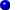Extinction angle

This is the angle formed by one line of the crystal with the extinction position. Either the longest dimension of the mineral or the system of cleavage lines are generally used as this line of reference.

In order to determine the angle, at first only the polariser is incorporated and the line is made to coincide with the direction of the polariser (E-W). The analyser is introduced and turned slowly until extinction occurs and the angle turned will be the extinction angle (movie 1 and movie 2). If the angle is greater than 45°, the analyser should be turned the other way to find the real angle. If when the analyser is introduced, the crystal is already dark, the extinction angle is 0° and the mineral is said to have a straight, or parallel extinction (movie 3).

The measurement of the extinction angle is of more importance if it is measured in relation to a certain direction of vibration, e.g. the slow component of the mineral.test

Index | Introduction | PPL | XPL orthos